Function Repository Resource:

SVGImport

Import an SVG file as a graphics object

Contributed by: Richard Hennigan (Wolfram Research)
 ResourceFunction["SVGImport"][path] imports the SVG file at path as a Graphics object. ResourceFunction["SVGImport"][ByteArray[…]] imports the given byte array as contents of an SVG file. ResourceFunction["SVGImport"]["string"] imports the given string as contents of an SVG file.

Details and Options

Possible values for path are:
 "path" a string corresponding to a file path or URL File [ … ] a File object URL [ … ] a URL object LocalObject [ … ] CloudObject [ … ]
ResourceFunction["SVGImport"] has the following options:
 "ShowWarnings" whether to show warnings about potential conversion inaccuracies "IntermediateFormat" an intermediate file format to be used during conversion

Examples

Basic Examples (4)

Import an SVG file as vector graphics:

 In:=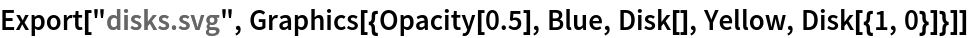Out=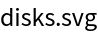In:=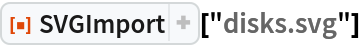Out=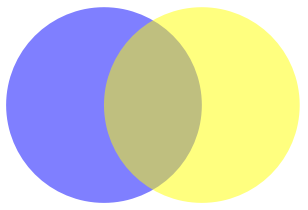Import an SVG with formatted text:

 In:=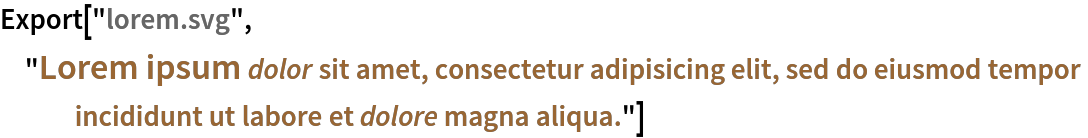Out=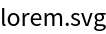In:=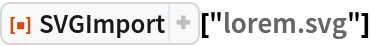Out=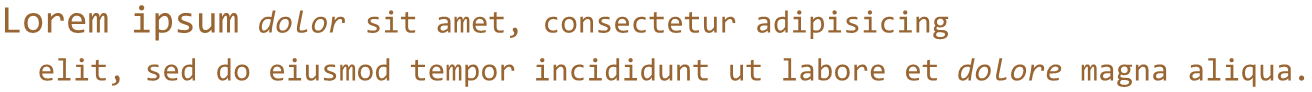Import an SVG from the web:

 In:=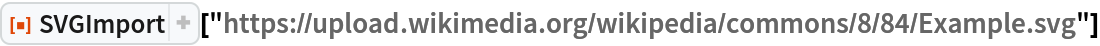Out=Import a formula:

 In:=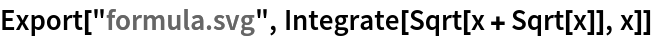Out=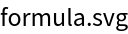In:=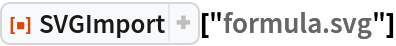Out=Scope (5)

Import an SVG from a string:

 In:=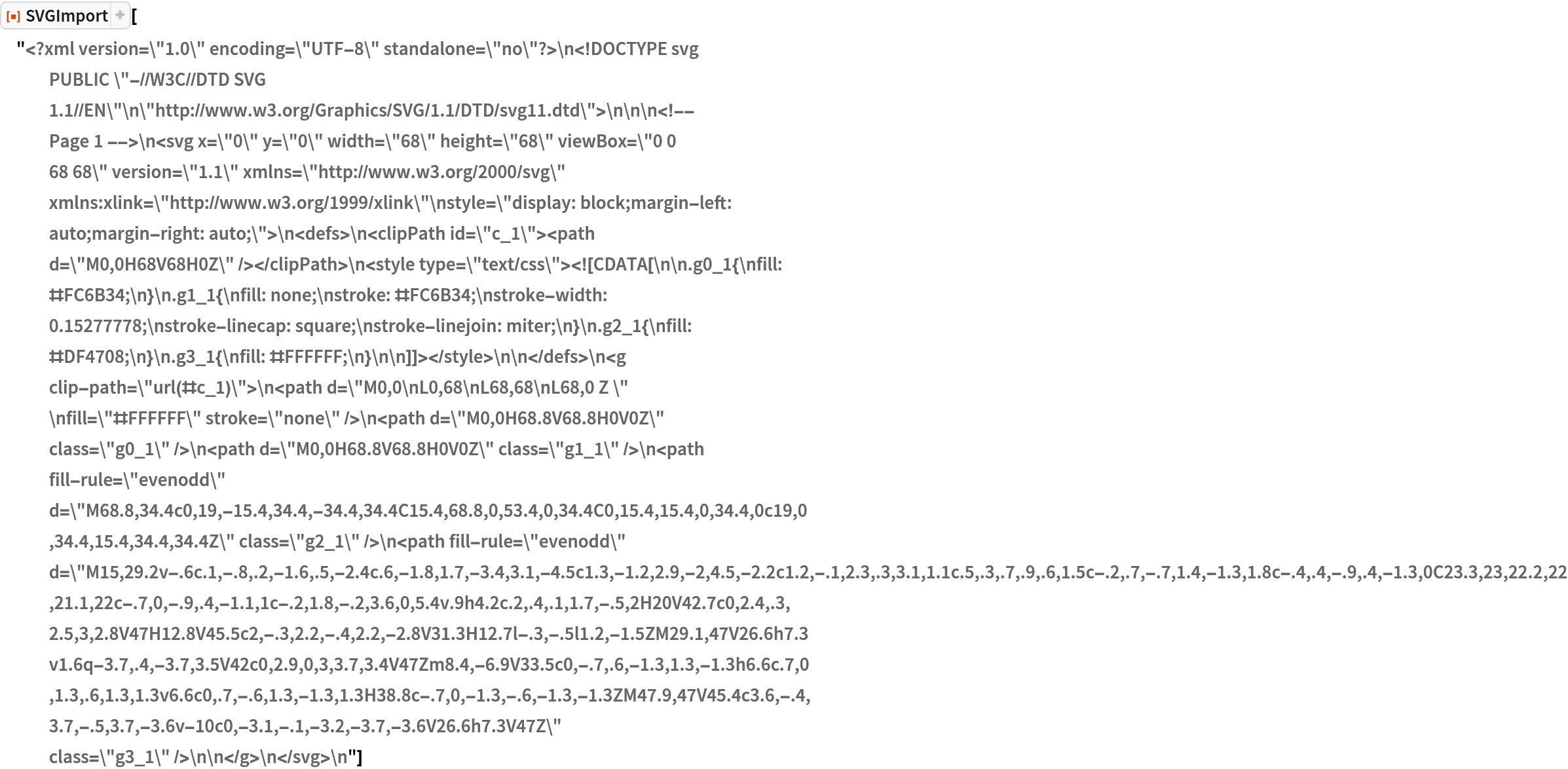Out=Import from a byte array:

 In:=Out=Import from a URL:

 In:=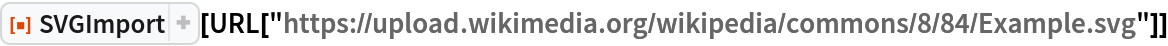Out=Import a CloudObject:

 In:=Out=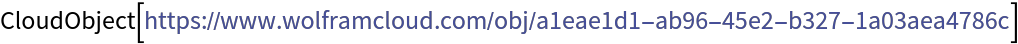In:=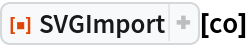Out=Import a LocalObject:

 In:=Out=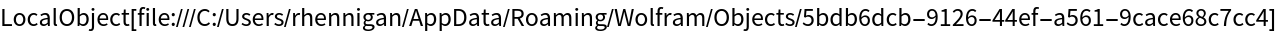In:=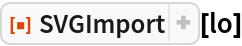Out=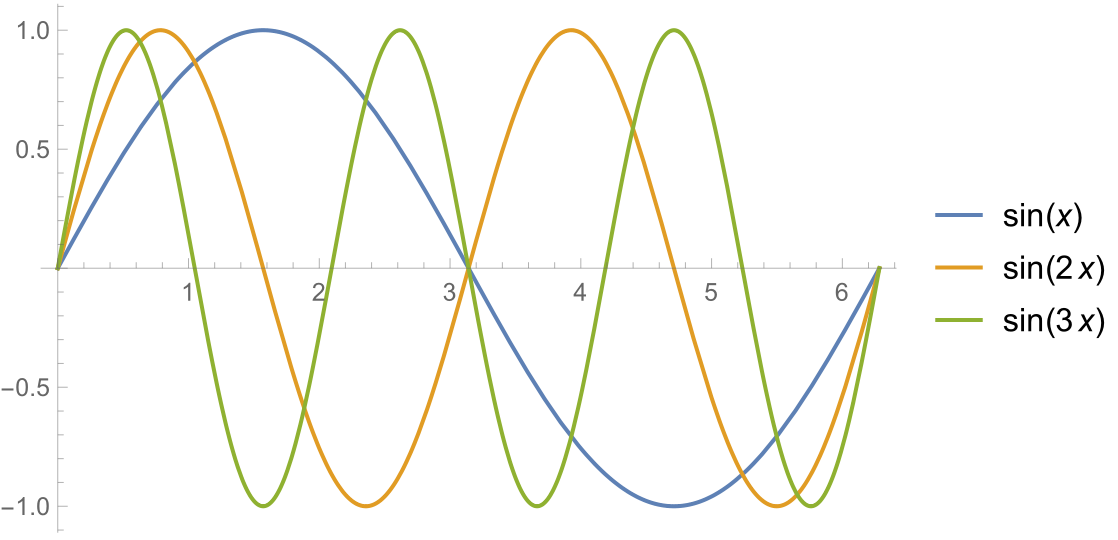Options (6)

ShowWarnings (3)

By default, warnings about potential conversion issues are not shown. In this example, some complex gradients were not converted correctly:

 In:=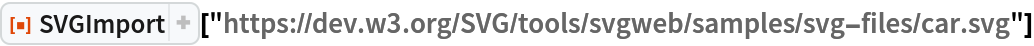Out=See the expected appearance:

 In:=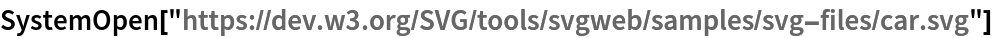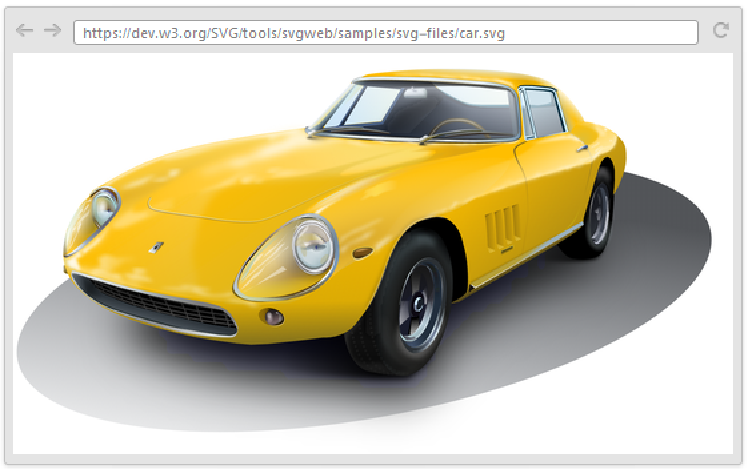Show warnings to see details about conversion problems:

 In:=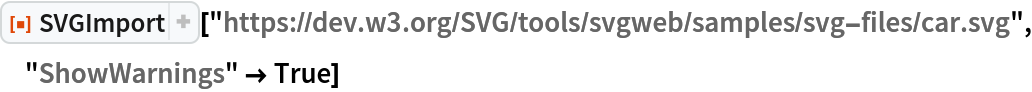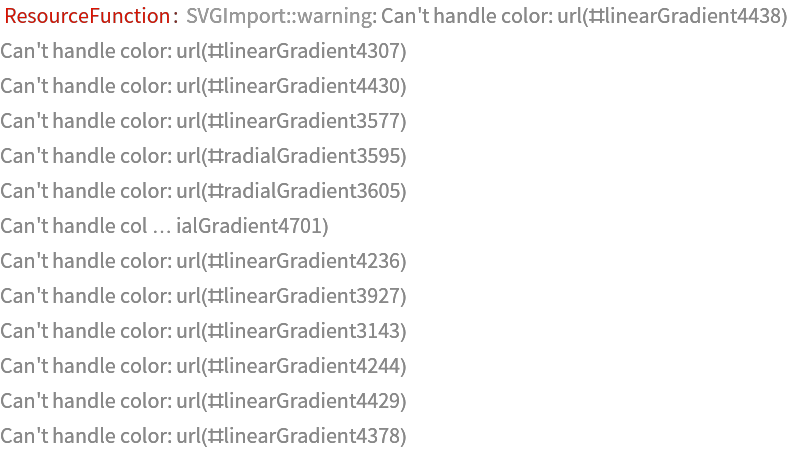Out=IntermediateFormat (3)

By default, SVGImport uses "PDF" as an intermediate format, which sometimes encounters issues with gradients:

 In:=Out=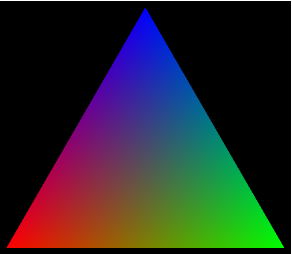In:=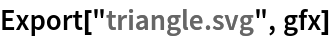Out=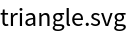In:=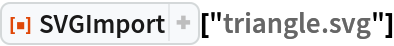Out=Using "PNG" as the intermediate format can improve results in some cases:

 In:=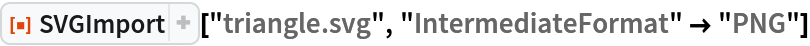Out=The result will no longer be scalable vector graphics, however:

 In:=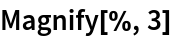Out=Properties and Relations (2)

"SVG" is a supported format for Export, but not Import:

 In:=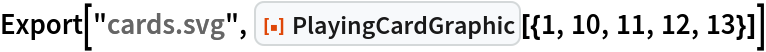Out=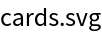In:=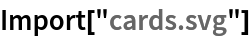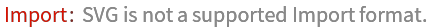Out=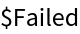Use SVGImport to import SVG files:

 In:=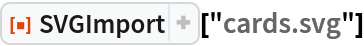Out=SVG files contain scalable vector graphics, so the results of SVGImport are scalable as well:

 In:=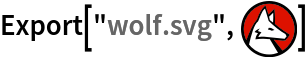Out=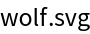In:=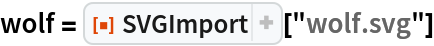Out=In:=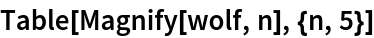Out=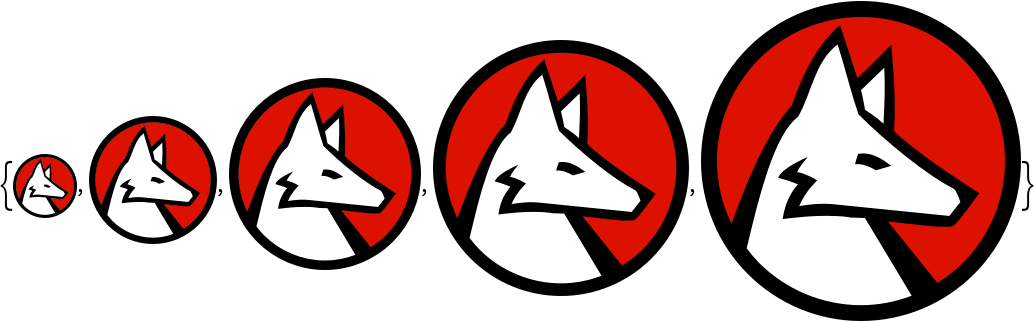Possible Issues (2)

Dynamic JavaScript content will not work:

 In:=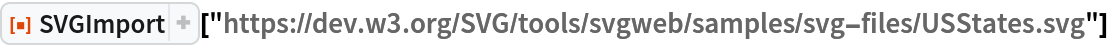Out=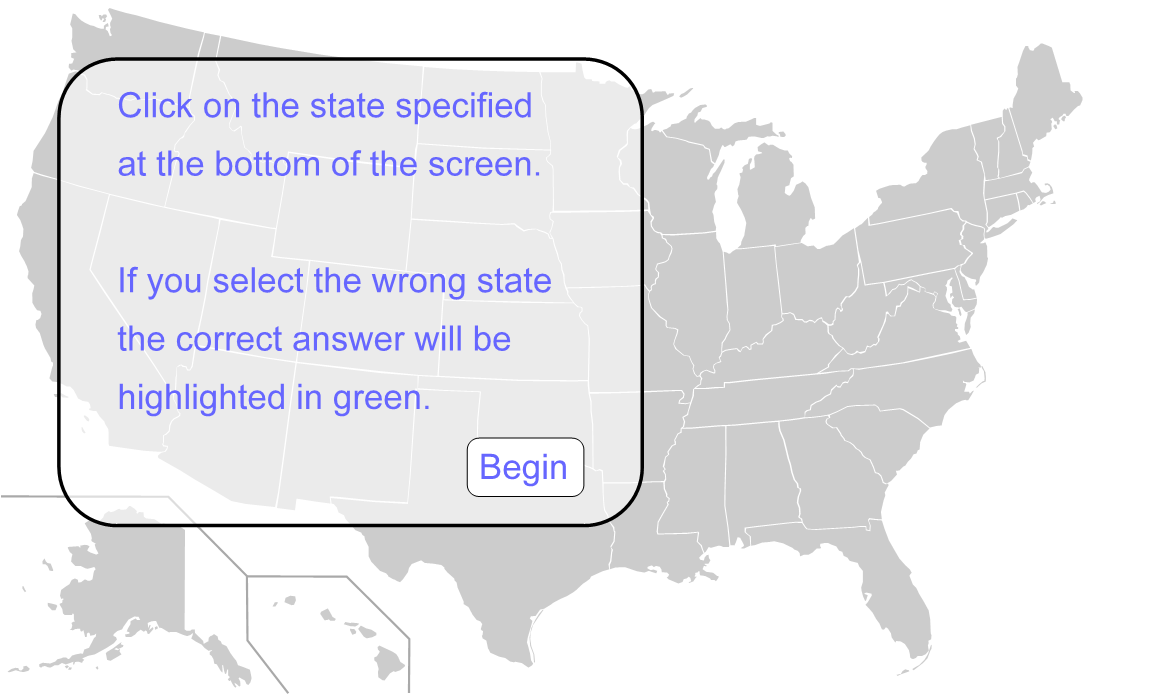Animations are also not supported:

 In:=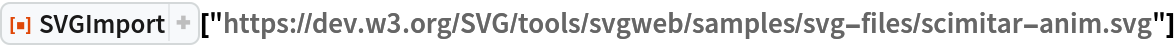Out=Author Notes

Change log

1.0.2: 2021-01-21

Fixed:

Fixed an issue with Python executables not being found in 12.2 due to a change in FindExternalEvaluators.